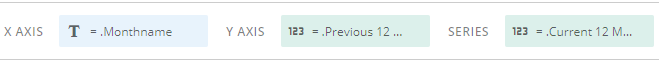# Re: Period over Period using stacked bars

I was looking to add an extra layer of granularity to my period over period chart.  Basically I want to combine a Year over Year card, and a stacked bar so we can quickly see that the balance between the two components isn't changing.  With this chart I'm just looking to see the quantities, and I'm not going to be digging into variance YoY.

Thanks for the help on this!

• So if you're wanting to do comparison between objects over the same timescale (ie, YoY by month) I would personally use something like a grouped bar instead of stacked. I think it's a bit easier to tell if there is a difference between values.

Here's how I would tackle it:

1.) Create a Monthname beastmode to return on the month name (this will be your X-axis)

MONTHNAME(`Date`)

2.) Create a Date Orderer beastmode so that it orders your month names correctly and drop this into your sorting field

CASE WHEN MONTH(CURDATE()) = MONTH(`Date`) THEN 1
WHEN MONTH(DATE_SUB(CURDATE(), interval 1 MONTH)) = MONTH(`Date`) THEN 2
WHEN MONTH(DATE_SUB(CURDATE(), interval 2 MONTH)) = MONTH(`Date`) THEN 3
WHEN MONTH(DATE_SUB(CURDATE(), interval 3 MONTH)) = MONTH(`Date`) THEN 4
WHEN MONTH(DATE_SUB(CURDATE(), interval 4 MONTH)) = MONTH(`Date`) THEN 5
WHEN MONTH(DATE_SUB(CURDATE(), interval 5 MONTH)) = MONTH(`Date`) THEN 6
WHEN MONTH(DATE_SUB(CURDATE(), interval 6 MONTH)) = MONTH(`Date`) THEN 7
WHEN MONTH(DATE_SUB(CURDATE(), interval 7 MONTH)) = MONTH(`Date`) THEN 8
WHEN MONTH(DATE_SUB(CURDATE(), interval 8 MONTH)) = MONTH(`Date`) THEN 9
WHEN MONTH(DATE_SUB(CURDATE(), interval 9 MONTH)) = MONTH(`Date`) THEN 10
WHEN MONTH(DATE_SUB(CURDATE(), interval 10 MONTH)) = MONTH(`Date`) THEN 11
WHEN MONTH(DATE_SUB(CURDATE(), interval 11 MONTH)) = MONTH(`Date`) THEN 12
END

3. Now you will create each of the "bars" of your graph. If you're just doing a count on current year vs previous year...

Current Year

COUNT(CASE WHEN `Date` IS NOT NULL AND  and `Date` >= DATE_SUB(DATE_FORMAT(CURDATE(), '%Y-%m-01'),interval 11 month)  THEN `ItemNumber`

END)

Previous Year

COUNT(CASE WHEN
`Date` <= LAST_DAY(DATE_SUB(CURDATE(), interval 12 month))
AND
`Date` >= DATE_SUB(DATE_FORMAT(CURDATE(), '%Y-%m-01'), interval 23 month)
THEN
`ItemNum`

END)

Once those are done, your Axis option should look like the following:From this point you can modify/add additional columns where you edit your Case statements to limit by specific items you want to compare (ie, AND `itemtype` = 'A')

Hopefully this will get you on started on the right track. Let me know if you have any other questions.

Sincerely,

ValiantSpur

**Please mark "Accept as Solution" if this post solves your problem
**Say "Thanks" by clicking the "heart" in the post that helped you.

This discussion has been closed.